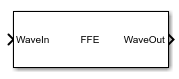# FFE

Models a feed-forward equalizer

Since R2019a

•Libraries:
SerDes Toolbox / Datapath Blocks

## Description

The FFE block applies a feed-forward equalizer (FFE) as a symbol-spaced finite-impulse response (FIR) filter to a sample-by-sample input signal or an impulse response vector input signal. This filtering reduces distortions due to channel loss impairments.

## Ports

### Input

expand all

Input baseband signal. The input signal can be a sample-by-sample signal specified as a scalar, or an impulse response vector signal.

Data Types: `double`

### Output

expand all

Filtered channel output. If the input signal is a sample-by-sample signal specified as a scalar, the output is also scalar. If the input signal is an impulse response vector signal, the output is also a vector.

Data Types: `double`

## Parameters

expand all

FFE operating mode:

• `Off`FFE is bypassed and the input waveform remains unchanged.

• `Fixed`FFE applies the FFE tap weights specified in Tap weights to input waveform.

#### Programmatic Use

• Use `get_param(gcb,'Mode')` to view the current FFE Mode.

• Use `set_param(gcb,'Mode',value)` to set FFE to a specific Mode.

FFE tap weights, specified as a row vector in volts. The length of the vector specifies the number of taps. The vector element value specifies the strength of the tap at that element position. The tap with the largest magnitude is the main tap and therefore defines the number of pre- and post-cursor taps.

You can use a valid MATLAB expression to evaluate the Initial tap weights (V) row vector.

Example: `set_param(gcb,'TapWeights',"zeros[(1,4) 1 zeros(1,15)]")` creates an FFE with 20 taps where the fifth tap is the main tap.

#### Programmatic Use

• Use `get_param(gcb,'TapWeights')` to view the current FFE Tap weights.

• Use `set_param(gcb,'TapWeights',value)` to set FFE to a specific Tap weights vector.

Data Types: `double`

Select to normalize tap weight vectors so that the sum of the absolute values of the Tap weights vector elements is one.

Click to plot the response of the FFE FIR (finite impulse response) filter.

IBIS-AMI parameters

Select to include Mode as a parameter in the IBIS-AMI file. If you deselect Mode, it is removed from the AMI files, effectively hard-coding Mode to its current value.

Select to include Tap weights as a parameter in the IBIS-AMI file. If you deselect Tap weights, it is removed from the AMI files, effectively hard-coding Tap weights to its current value.

## Version History

Introduced in R2019a